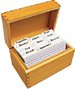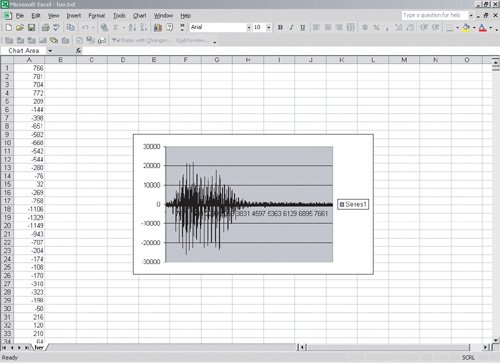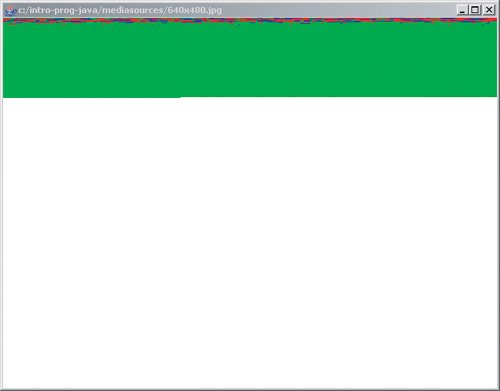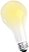# Section 12.6. Using Text to Shift Between Media

[Page 427 (continued)]

### 12.6. Using Text to Shift Between Media

As we said at the beginning of this chapter, we can think about text as the unimedia. We can map from sound to text and back again, and the same with pictures. And more interestingly, we can go from sound to text ... to pictures!

Why would we want to do any of this? Why should we care about transforming media in this way? For the same reasons that we care about digitizing media at all. Digital media transformed into text can be more easily transmitted from place to place, checked for errors, and even corrected for errors. It turns out that very often when you are attaching binary files to an e-mail message, your binary file is actually converted to text first! In general, choosing a new representation allows you to do new things.

Mapping sound to text is easy. Sound is just a series of samples (numbers). We can easily write these out to a file. Let's add a method to do this to the Sound class. We will need to convert the number to a string. We can use the method valueOf on the String class to do this. This is a class (static) method so it can be invoked using String.valueOf.

[Page 428]

##### Program 113. Write a Sound to a File as Text Numbers`/** * Method to write out the values in the sound to a file * as text * @param fileName the name of the file to write to */ public void writeSamplesAsText(String fileName) { int value = 0; // try the following try { // try to open the buffered writer BufferedWriter writer = new BufferedWriter(new FileWriter(fileName)); // loop through the samples for (int i = 0; i < this.getLength(); i++) { // get the int value value = this.getSampleValueAt(i); // write it as a string (text) writer.write(String.valueOf(value)); // add the new line writer.newLine(); } // close the writer writer.close(); } catch (Exception ex) { SimpleOutput.showError("Error during write"); ex.printStackTrace(); } }`

To run this we can use the following main method in the Sound class.

`public static void main(String[] args) {   Sound s = new Sound(FileChooser.getMediaPath("her.wav"));   s.writeSamplesAsText(FileChooser.getMediaPath("her.txt")); }`

After we have compiled the Sound class we can run it using:

`> java Sound`

[Page 429]

#### How it Works

We're taking the file name to write to as a parameter. We open the file for writing and then we loop though each sample, converting it to a string and writing the string to a file. We use the newLine method of BufferedReader to add a new line after we write each sample value as a string.

What can we do with sound values written as text? We can manipulate it as a series of numbers, such as with Excel (Figure 12.6)! We can open Excel and open the file. We can tell it the delimiters are spaces and it will read the numbers into Column A. Once we have the data in Excel we can do modifications, such as multiplying each sample by 2.0.

##### Figure 12.6. Sound-as-text file read into Excel.We can even graph the numbers, and see the same kind of sound graph as we've seen in MediaTools (Figure 12.7). Select the column of numbers and then click on INSERT and CHART. You can just click on the Finish button to use the defaults. (You might get an error, thoughExcel doesn't like to graph more than 32,000 points, and even at 22,000 samples per second, that's not a lot of samples.)

##### Figure 12.7. Sound-as-text file graphed in Excel. (This item is displayed on page 430 in the print version)How do we convert a series of numbers back into a sound? Say that you do some modification to the numbers in Excel, and now you want to hear the result. How do you do it? The mechanics in Excel are easy: Simply copy the column you want into a new worksheet, save it as text, then get the pathname of the text file to use in Java.

The program itself is a little more complicated. When going from sound to text, we knew that we could use getSampleValueAt(i) to write out all the samples. But how do we know how many lines are in the file? We don't really know until we have read them all in. We have to watch out for two problems: (a) having more lines in the file than we can fit into the sound that we're using to read into, and (b) running out of lines before we reach the end of the sound.

[Page 430]

So, for our text-to-sound example, we want to keep reading samples from the file and storing them into the sound as long as we have numbers in the file and as long as there is still room in the sound. We use the method Integer.parseInt to convert the string number into an integer number.

`> System.out.println("1234" + 5); 12345 > System.out.println(Integer.parseInt("1234") + 5); 1239`

Does the new method that we are writing work with a current Sound object? No, it creates a Sound object so it can be a class (static) method.

##### Program 114. Convert a File of Text Numbers into a Sound (This item is displayed on pages 430 - 431 in the print version)`/** * Method to create a sound from a text file * @param fileName the name of the file to read from * @return the created sound object */ public static Sound createSoundFromTextFile(String fileName) { String line = null; int value = 0; // create the sound to read into Sound s = new Sound(FileChooser.getMediaPath("sec3silence.wav")); [Page 431] // try the following try { // create the buffered reader BufferedReader reader = new BufferedReader(new FileReader(fileName)); // loop reading the values int index = 0; while ((line = reader.readLine()) != null && index < s.getLength()) { value = Integer.parseInt(line); s.setSampleValueAt(index++, value); } // close the reader reader.close(); } catch (FileNotFoundException ex) { SimpleOutput.showError("Couldn't find file " + fileName); fileName = FileChooser.pickAFile(); s = createSoundFromTextFile(fileName); } catch (Exception ex) { SimpleOutput.showError("Error during read or write"); ex.printStackTrace(); } return s; }`

#### How it Works

The method createSoundFromTextFile takes a filename as input that contains the samples as text. We open up a silent three-second sound to hold the sound. Next we create a BufferedReader to read the file. We loop reading the file until either the end of the file is reached or the index is equal to the length of the sound. In the body of the loop we convert the string to an integer and then set the sample value at the current index. We increment the current index. When we are done we return the created Sound object.

But we don't have to map from sounds to text and back to sounds. We could map a sound to a picture instead! The below program takes a sound and maps each sample to a pixel. All we have to do is to define our mapping, how we want to represent the samples. We chose a very simple one: If the sample is greater than 1,000, the pixel is red; less than -1,000 is blue, everything else is green (Figure 12.8).

##### Figure 12.8. A visualization of the sound "her.wav''. (This item is displayed on page 432 in the print version)We also have to deal with the case where we run out of samples before we run out of pixels. We can add this to the continuation test of a for loop using 'and' (&&).

##### Program 115. Creating a Picture from a Sound (This item is displayed on pages 431 - 432 in the print version)`/** * Method to turn a sound into a picture * @return a created picture */ [Page 432]public Picture createPicture() { int value = 0; Pixel pixel = null; // create a picture to write to Picture p = new Picture(FileChooser.getMediaPath("640x480.jpg")); // loop through the pixels Pixel[] pixelArray = p.getPixels(); // loop through the pixels for (int i = 0; i < pixelArray.length && i < this.getLength(); i++) { // get this pixel pixel = pixelArray[i]; // set the color based on the sample value value = this.getSampleValueAt(i); if (value > 1000) pixel.setColor(Color.RED); else if (value < -1000) pixel.setColor(Color.BLUE); else pixel.setColor(Color.GREEN); } return p; }`

[Page 433]

#### How it Works

In createPicture(), we open up a 640x480 blank picture. We loop while i is less than the number of pixels in the picture and also less than the samples in the current sound. Each time through the loop we get a sample value at i and figure out a mapping to a color, then set the pixel value to that color. Then we increment i. We return the created Picture object at the end of the method.

Think about how WinAmp does its visualizations, or how Excel or MediaTools graph, or how this program does its visualization. Each is just deciding a different way of mapping from samples to colors and space. It's just a mapping. It's all just bits.## Computer Science Idea: It's All Just Bits

Sound, pictures, and text are all just "bits.'' They're just information. We can map from one to the other as we wish. We merely have to define our representation.

A really smart mathematician, Kurt Gödel, used the notion of encodings to come up with one of the most brilliant proofs of the twentieth century. He proved the Incompleteness Theorem, in which he proved that any powerful mathematical system cannot prove all mathematical truths. He figured out a mapping from mathematical statements of truth to numbers. This was long before we had ASCII, which maps numbers to characters. Once the mathematical statements were numbers, he was able to show that there are numbers representing true statements that could not be derived from the mathematical system. In this way, he showed that no system of logic can prove all true statements. By changing his encoding, he gained new capabilities, and thus was able to prove something that no one knew before.Introduction to Computing & Programming Algebra in Java(c) A Multimedia Approach
ISBN: N/A
EAN: N/A
Year: 2007
Pages: 191